# “Locally of finite type” is a local property

Previously we showed that morphisms locally of finite type are preserved under base change. We can use this to show thatGiven a morphism of schemes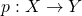, the preimage of any affinecan be covered by affines such that the corresponding ring maps are of finite type.

Alternatively, if we define a morphism locally of finite type to be one that satisfies, then what we are saying is that such a property can be checked on a cover; we can replace “any affine” with “an affine in a cover of affines”.

Let’s try to prove. First, we base change to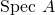. Since the morphism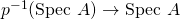is also locally of finite type, we can coverby affines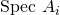such that their preimages can be covered by the spectra of finitely-generated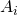-algebras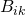. However, we don’t know if these are finitely-generated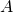-algebras! To fix this, we base change to even smaller affines. Coverby basic open sets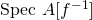. This gives us a cover of each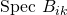by basic open sets of the form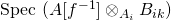. Since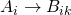is of finite type,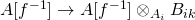is of finite type. Sinceis clearly of finite type,is of finite type, giving us the desired cover of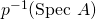. The following diagram may be illustrative (every square is a pullback)

# Group schemes and graded rings

In this post we will describe how an action of the multiplicative group scheme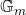on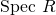defines a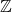-grading of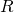. A future post may describe how this relates to projective schemes. (I will do all of this using diagrams, but there may be some easier way using the functors of points). All this was taught to me by Mark Haiman in Math 256B (Algebraic Geometry) at UC Berkeley.

Fix a field; we will work in the category of-schemes. Thuswill be a-algebra, and we will establish a graded-algebra structure on. However, none of our arguments change if we just letbe. A group scheme is a group object in the category of-schemes. A precise definition can be found here. Most importantly, group schemes can act on other schemes. The definition of a group scheme action can be found here. Note that all definitions are given by diagrams (or functor of points). For example, we specify the “identity element” of a group scheme by a map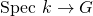, rather than selecting some point in the underlying topological space.is defined as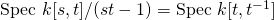. (for shorthand, we will writeas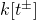). As a variety, it can be thought of as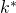, the “punctured affine line”. Its group operation is given by a map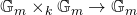which corresponds to the-algebra map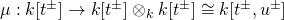defined by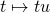. The identity is given by a map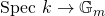corresponding to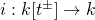defined by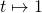.

Supposeacts on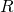. The action mapcorresponds to a-algebra map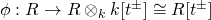such that the following diagrams commute:

Associativity: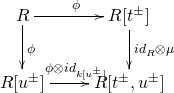Identity: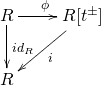For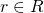, write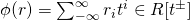, where almost all the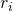are zero. Then the first diagram implies thatif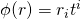(i.e. the polynomial is just a single monomial), then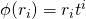.

This is because, along the top and right arrows, we have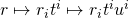and along the left and bottom arrows we have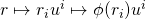. Furthermore, the second diagram says that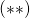for all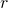,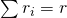.

Therefore, letting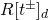stand for the degree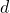homogenous component of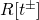(so that it consists of multiples of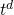), let. Since all theare disjoint, their preimages are disjoint as well. Furthermore, for an arbitrary element, we have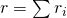by, and by, we have that each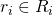. Thus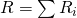as a direct sum.

It remains to show that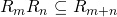. But this is easy: if, then, so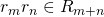as desired.